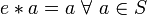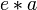# Semigroup with left neutral element where every element is left-invertible equals group

Jump to: navigation, search

## Statement

Suppose$(S,*)$ is a semigroup containing an element$e$ satisfying the following two conditions:

1.$e$ is a left neutral element for$S$, i.e.,$e * a = a \ \forall \ a \in S$
2. For all$a \in S$, there exists$b \in S$ such that$b * a = e$.

Then,$S$ is a group under$*$ with$e$ as the identity element and the two-sided inverse of$a$ being the unique$b$ that satisfies condition (2).

## Facts used

1. Monoid where every element is left-invertible equals group

## Proof

This proof uses a tabular format for presentation. Provide feedback on tabular proof formats in a survey (opens in new window/tab) | Learn more about tabular proof formats|View all pages on facts with proofs in tabular format

Thanks to Fact (1), it suffices to prove that$S$ is a monoid with two-sided identity element (neutral element)$e$ -- Fact (1) does the rest of our work.

Given:$(S,*)$ is a semigroup containing an element$e$ satisfying the following two conditions:

1.$e$ is a left neutral element for$S$, i.e.,$e * a = a \ \forall \ a \in S$
2. For all$a \in S$, there exists$b \in S$ such that$b * a = e$.

To prove: For any element$a \in S$, we have$a * e = a$.

Proof: We fix the element$a$ for the proof.

Step no. Assertion/construction Facts used Given data used Previous steps used Explanation
1 There exists an element$b \in S$ such that$b * a = e$. follows from condition (2)
2 There exists an element$c \in S$ such that$c * b = e$. follows from condition (2) applied to$b$ Step (1)
3 The product$(c * b) * (a * e)$ simplifies to$a * e$ Step (2) We use$c * b = e$ from Step (2) and then use that$e$ is left neutral
4 The product$c * ((b * a) * e)$ simplifies to$c * e$ Step(1) Using$b * a = e$, the product becomes$c * (e * e)$. Using that$e$ is left neutral, we get$e * e = e$, so the expression simplifies to$c * e$.
5$c * e = a$ Steps (1), (2) We write$e = b * a$ and get$c * e = c * (b * a)$. Use associativity to rewrite as$(c * b) * a$. Now use$c * b = e$ to rewrite as$e * a$. Finally, use$e$ is left neutral to simplify to$a$.
6$c * b * a * e$ simplifies both to$a * e$ and to$a$, hence$a * e = a$. Steps (3), (4), (5) Step (3) simplifies one parenthesization of the product to$a * e$. Steps (4) and (5) simplify another parenthesization of the product to$a$. Thus, we are done.

Note that a little more work with the same steps would also show that$a = c$ and so$b$ is a two-sided inverse, completing the proof directly instead of invoking Fact (1). There's no substantive difference between completing the proof directly and invoking Fact (1), which in any case uses the same construction albeit in a simpler setting.